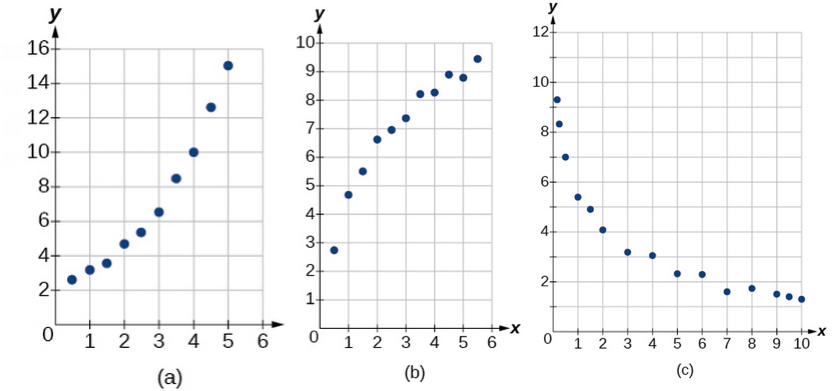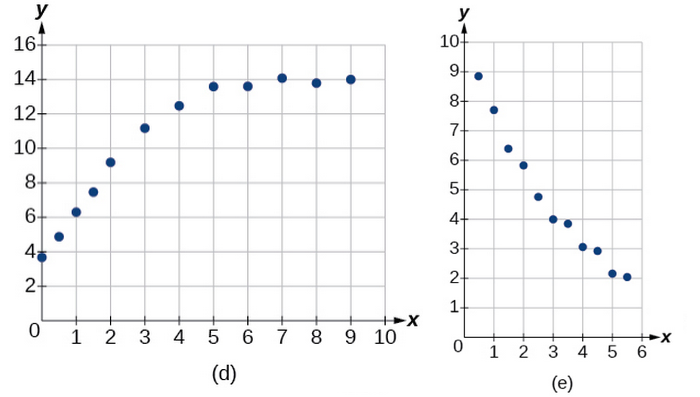## Section Exercises

1. What situations are best modeled by a logistic equation? Give an example, and state a case for why the example is a good fit.

2. What is a carrying capacity? What kind of model has a carrying capacity built into its formula? Why does this make sense?

3. What is regression analysis? Describe the process of performing regression analysis on a graphing utility.

4. What might a scatterplot of data points look like if it were best described by a logarithmic model?

5. What does the y-intercept on the graph of a logistic equation correspond to for a population modeled by that equation?

For the following exercises, match the given function of best fit with the appropriate scatterplot. Answer using the letter beneath the matching graph.6. $y=10.209{e}^{-0.294x}$

7. $y=5.598 - 1.912\mathrm{ln}\left(x\right)$

8. $y=2.104{\left(1.479\right)}^{x}$

9. $y=4.607+2.733\mathrm{ln}\left(x\right)$

10. $y=\frac{14.005}{1+2.79{e}^{-0.812x}}$

11. To the nearest whole number, what is the initial value of a population modeled by the logistic equation $P\left(t\right)=\frac{175}{1+6.995{e}^{-0.68t}}$? What is the carrying capacity?

12. Rewrite the exponential model $A\left(t\right)=1550{\left(1.085\right)}^{x}$ as an equivalent model with base e. Express the exponent to four significant digits.

13. A logarithmic model is given by the equation $h\left(p\right)=67.682 - 5.792\mathrm{ln}\left(p\right)$. To the nearest hundredth, for what value of p does $h\left(p\right)=62$?

14. A logistic model is given by the equation $P\left(t\right)=\frac{90}{1+5{e}^{-0.42t}}$. To the nearest hundredth, for what value of t does $P\left(t\right)=45$?

15. What is the y-intercept on the graph of the logistic model given in the previous exercise?

For the following exercises, use this scenario: The population P of a koi pond over x months is modeled by the function $P\left(x\right)=\frac{68}{1+16{e}^{-0.28x}}$.

16. Graph the population model to show the population over a span of 3 years.

17. What was the initial population of koi?

18. How many months will it take before there are 20 koi in the pond?

19. Use the intersect feature to approximate the number of months it will take before the population of the pond reaches half its carrying capacity.

For the following exercises, use this scenario: The population P of an endangered species habitat for wolves is modeled by the function $P\left(x\right)=\frac{558}{1+54.8{e}^{-0.462x}}$, where x is given in years.

20. Graph the population model to show the population over a span of 10 years.

21. What was the initial population of wolves transported to the habitat?

22. How many wolves will the habitat have after 3 years?

23. How many years will it take before there are 100 wolves in the habitat?

24. Use the intersect feature to approximate the number of years it will take before the population of the habitat reaches half its carrying capacity.

For the following exercises, refer to the table below.

 x f(x) 1 1125 2 1495 3 2310 4 3294 5 4650 6 6361

25. Use a graphing calculator to create a scatter diagram of the data.

26. Use the regression feature to find an exponential function that best fits the data in the table.

27. Write the exponential function as an exponential equation with base e.

28. Graph the exponential equation on the scatter diagram.

29. Use the intersect feature to find the value of x for which $f\left(x\right)=4000$.

For the following exercises, refer to the table below.

 x f(x) 1 555 2 383 3 307 4 210 5 158 6 122

30. Use a graphing calculator to create a scatter diagram of the data.

31. Use the regression feature to find an exponential function that best fits the data in the table.

32. Write the exponential function as an exponential equation with base e.

33. Graph the exponential equation on the scatter diagram.

34. Use the intersect feature to find the value of x for which $f\left(x\right)=250$.

For the following exercises, refer to the table below.

 x f(x) 1 5.1 2 6.3 3 7.3 4 7.7 5 8.1 6 8.6

35. Use a graphing calculator to create a scatter diagram of the data.

36. Use the LOGarithm option of the REGression feature to find a logarithmic function of the form $y=a+b\mathrm{ln}\left(x\right)$ that best fits the data in the table.

37. Use the logarithmic function to find the value of the function when = 10.

38. Graph the logarithmic equation on the scatter diagram.

39. Use the intersect feature to find the value of x for which $f\left(x\right)=7$.

For the following exercises, refer to the table below.

 x f(x) 1 7.5 2 6 3 5.2 4 4.3 5 3.9 6 3.4 7 3.1 8 2.9

40. Use a graphing calculator to create a scatter diagram of the data.

41. Use the LOGarithm option of the REGression feature to find a logarithmic function of the form $y=a+b\mathrm{ln}\left(x\right)$ that best fits the data in the table.

42. Use the logarithmic function to find the value of the function when = 10.

43. Graph the logarithmic equation on the scatter diagram.

44. Use the intersect feature to find the value of x for which $f\left(x\right)=8$.

For the following exercises, refer to the table below.

 x f(x) 1 8.7 2 12.3 3 15.4 4 18.5 5 20.7 6 22.5 7 23.3 8 24 9 24.6 10 24.8

45. Use a graphing calculator to create a scatter diagram of the data.

46. Use the LOGISTIC regression option to find a logistic growth model of the form $y=\frac{c}{1+a{e}^{-bx}}$ that best fits the data in the table.

47. Graph the logistic equation on the scatter diagram.

48. To the nearest whole number, what is the predicted carrying capacity of the model?

49. Use the intersect feature to find the value of x for which the model reaches half its carrying capacity.
For the following exercises, refer to the table below.

 x f(x) 0 12 2 28.6 4 52.8 5 70.3 7 99.9 8 112.5 10 125.8 11 127.9 15 135.1 17 135.9

50. Use a graphing calculator to create a scatter diagram of the data.

51. Use the LOGISTIC regression option to find a logistic growth model of the form $y=\frac{c}{1+a{e}^{-bx}}$ that best fits the data in the table.

52. Graph the logistic equation on the scatter diagram.

53. To the nearest whole number, what is the predicted carrying capacity of the model?

54. Use the intersect feature to find the value of x for which the model reaches half its carrying capacity.

55. Recall that the general form of a logistic equation for a population is given by $P\left(t\right)=\frac{c}{1+a{e}^{-bt}}$, such that the initial population at time t = 0 is $P\left(0\right)={P}_{0}$. Show algebraically that $\frac{c-P\left(t\right)}{P\left(t\right)}=\frac{c-{P}_{0}}{{P}_{0}}{e}^{-bt}$.

56. $\frac{c-P\left(t\right)}{P\left(t\right)}=\frac{c-\frac{c}{1+a{e}^{-bt}}}{\frac{c}{1+a{e}^{-bt}}}=\frac{\frac{c\left(1+a{e}^{-bt}\right)-c}{1+a{e}^{-bt}}}{\frac{c}{1+a{e}^{-bt}}}=\frac{\frac{c\left(1+a{e}^{-bt}-1\right)}{1+a{e}^{-bt}}}{\frac{c}{1+a{e}^{-bt}}}=1+a{e}^{-bt}-1=a{e}^{-bt}$

57. Working with the right side of the equation we show that it can also be rewritten as $a{e}^{-bt}$. But first note that when t = 0, ${P}_{0}=\frac{c}{1+a{e}^{-b\left(0\right)}}=\frac{c}{1+a}$. Therefore,

58. Use a graphing utility to find an exponential regression formula $f\left(x\right)$ and a logarithmic regression formula $g\left(x\right)$ for the points $\left(1.5,1.5\right)$ and $\left(8.5,\text{ 8}\text{.5}\right)$. Round all numbers to 6 decimal places. Graph the points and both formulas along with the line = x on the same axis. Make a conjecture about the relationship of the regression formulas.

59. Verify the conjecture made in the previous exercise. Round all numbers to six decimal places when necessary.

60. Find the inverse function ${f}^{-1}\left(x\right)$ for the logistic function $f\left(x\right)=\frac{c}{1+a{e}^{-bx}}$. Show all steps.

61. Use the result from the previous exercise to graph the logistic model $P\left(t\right)=\frac{20}{1+4{e}^{-0.5t}}$ along with its inverse on the same axis. What are the intercepts and asymptotes of each function?